### 3.3 Conditions

This section of the file defines parameters and boundary conditions which define the nature of the problem to be solved. These are enclosed in the `CONDITIONS` tag.

#### 3.3.1 Parameters

Numerical parameters may be required by a particular solver (for instance time-integration or physical parameters), or may be arbitrary and only used for the purpose of simplifying the problem specification in the session file (e.g. parameters which would otherwise be repeated in the definition of an initial condition and boundary conditions). All parameters are enclosed in the `PARAMETERS` XML element.

1<PARAMETERS>
2    ...
3</PARAMETERS>

A parameter may be of integer or real type and may reference other parameters defined previous to it. It is expressed in the file as

1<P> [PARAMETER NAME] = [PARAMETER VALUE] </P>

For example,

1<P> NumSteps = 1000              </P>
2<P> TimeStep = 0.01              </P>
3<P> FinTime  = NumSteps*TimeStep </P>

A number of pre-defined constants may also be used in parameter expressions, for example `PI`. A full list of supported constants is provided in Section 3.7.1.2.

#### 3.3.2 Time Integration Scheme

These specify properties to define the parameters specific to the time integration scheme to be used. The parameters are specified as XML elements and require a string corresponding to the time-stepping method and the order, and optionally the variant and free parameters. For example,

1 <TIMEINTEGRATIONSCHEME>
2   <METHOD> IMEX </METHOD>
3   <VARIANT> DIRK </VARIANT>
4   <ORDER> 2 </ORDER>
5   <FREEPARAMETERS> 2 3 </FREEPARAMETERS>
6 </TIMEINTEGRATIONSCHEME>

For additional details on the different time integration schemes refer to the developer’s guide.

#### 3.3.3 Solver Information

These specify properties to define the actions specific to solvers, typically including the equation to solve and the projection type. The property/value pairs are specified as XML attributes. For example,

1<SOLVERINFO>
3  <I PROPERTY="Projection"            VALUE="Continuous"           />
4</SOLVERINFO>

Boolean-valued solver properties are specified using `True` or `False`. The list of available solvers in Nektar++ can be found in Part III.

##### 3.3.3.1 Drivers

Drivers are defined under the `CONDITIONS` section as properties of the `SOLVERINFO` XML element. The role of a driver is to manage the solver execution from an upper level.

The default driver is called `Standard` and executes the following steps:

1. Prints out on screen a summary of all the conditions defined in the input file.
2. Sets up the initial and boundary conditions.
3. Calls the solver defined by `SolverType` in the `SOLVERINFO` XML element.
4. Writes the results in the output (.fld) file.

In the following example, the driver `Standard` is used to manage the execution of the incompressible Navier-Stokes equations:

1 <TIMEINTEGRATIONSCHEME>
2    <METHOD> IMEX </METHOD>
3    <ORDER> 2 </ORDER>
4 </TIMEINTEGRATIONSCHEME>
5
6 <SOLVERINFO>
8    <I PROPERTY="SolverType"            VALUE="VelocityCorrectionScheme" />
9    <I PROPERTY="Projection"            VALUE="Galerkin"                 />
10    <I PROPERTY="Driver"                VALUE="Standard"                 />
11</SOLVERINFO>

If no driver is specified in the session file, the driver `Standard` is called by default. Other drivers can be used and are typically focused on specific applications. As described in Sec. 11.3.1 and 11.4.1, the other possibilities are:

• `ModifiedArnoldi` - computes of the leading eigenvalues and eigenmodes using modified Arnoldi method.
• `Arpack` - computes of eigenvalues/eigenmodes using Implicitly Restarted Arnoldi Method (ARPACK).
• `SteadyState` - uses the Selective Frequency Damping method (see Sec. 11.1.5) to obtain a steady-state solution of the Navier-Stokes equations (compressible or incompressible).

#### 3.3.4 Variables

These define the number (and name) of solution variables. Each variable is prescribed a unique ID. For example a two-dimensional flow simulation may define the velocity variables using

1<VARIABLES>
2  <V ID="0"> u </V>
3  <V ID="1"> v </V>
4</VARIABLES>

#### 3.3.5 Global System Solution Algorithm

Many Nektar++ solvers use an implicit formulation of their equations to, for instance, improve timestep restrictions. This means that a large matrix system must be constructed and a global system set up to solve for the unknown coefficients. There are several approaches in the spectral/hp element method that can be used in order to improve efficiency in these methods, as well as considerations as to whether the simulation is run in parallel or serial. Nektar++ opts for ‘sensible’ default choices, but these may or may not be optimal depending on the problem under consideration.

This section of the XML file therefore allows the user to specify the global system solution parameters, which dictates the type of solver to be used for any implicit systems that are constructed. This section is particularly useful when using a multi-variable solver such as the incompressible Navier-Stokes solver, as it allows us to select different preconditioning and residual convergence options for each variable. As an example, consider the block defined by:

1<GLOBALSYSSOLNINFO>
2  <V VAR="u,v,w">
3    <I PROPERTY="GlobalSysSoln"             VALUE="IterativeStaticCond" />
4    <I PROPERTY="Preconditioner"            VALUE="LowEnergyBlock"/>
5    <I PROPERTY="IterativeSolverTolerance"  VALUE="1e-8"/>
6  </V>
7  <V VAR="p">
8    <I PROPERTY="GlobalSysSoln"             VALUE="IterativeStaticCond" />
9    <I PROPERTY="Preconditioner"     VALUE="FullLinearSpaceWithLowEnergyBlock"/>
10    <I PROPERTY="IterativeSolverTolerance"  VALUE="1e-6"/>
11  </V>
12</GLOBALSYSSOLNINFO>

The above section specifies that the variables `u,v,w` should use the `IterativeStaticCond` global solver alongside the `LowEnergyBlock` preconditioner and an iterative tolerance of 10-8 on the residuals. However the pressure variable `p` is generally stiffer: we therefore opt for a more expensive `FullLinearSpaceWithLowEnergyBlock` preconditioner and a larger residual of 10-6. We now outline the choices that one can use for each of these parameters and give a brief description of what they mean.

Defaults for all fields can be defined by setting the equivalent property in the `SOLVERINFO` section. Parameters defined in this section will override any options specified there.

##### 3.3.5.1 `GlobalSysSoln` options

Nektar++ presently implements four methods of solving a global system:

• Direct solvers construct the full global matrix and directly invert it using an appropriate matrix technique, such as Cholesky factorisation, depending on the properties of the matrix. Direct solvers only run in serial.
• Iterative solvers instead apply matrix-vector multiplications repeatedly, using the conjugate gradient method, to converge to a solution to the system. For smaller problems, this is typically slower than a direct solve. However, for larger problems it can be used to solve the system in parallel execution.
• Xxt solvers use the XXT library to perform a parallel direct solve. This option is only available if the `NEKTAR_USE_MPI` option is enabled in the CMake configuration.
• PETSc solvers use the PETSc library, giving access to a wide range of solvers and preconditioners. See section 3.3.5.4 below for some additional information on how to use the PETSc solvers. This option is only available if the `NEKTAR_USE_PETSC` option is enabled in the CMake configuration.

Warning: Both the Xxt and PETSc solvers are considered advanced and are under development – either the direct or iterative solvers are recommended in most scenarios.

These solvers can be run in one of three approaches:

• The Full approach constructs the global system based on all of the degrees of freedom contained within an element. For most of the Nektar++ solvers, this technique is not recommended.
• The StaticCond approach applies a technique called static condensation to instead construct the system using only the degrees of freedom on the boundaries of the elements, which reduces the system size considerably. This is the default option in parallel.
• MultiLevelStaticCond methods apply the static condensation technique repeatedly to further reduce the system size, which can improve performance by 25-30% over the normal static condensation method. It is therefore the default option in serial. Note that whilst parallel execution technically works, this is under development and is likely to be slower than single-level static condensation: this is therefore not recommended.

The `GlobalSysSoln` option is formed by combining the method of solution with the approach: for example `IterativeStaticCond` or `PETScMultiLevelStaticCond`.

##### 3.3.5.2 Preconditioner options

Preconditioners can be used in the iterative and PETSc solvers to reduce the number of iterations needed to converge to the solution. There are a number of preconditioner choices, the default being a simple Jacobi (or diagonal) preconditioner, which is enabled by default. There are a number of choices that can be enabled through this parameter, which are all generally discretisation and dimension-dependent:

 Name Dimensions Discretisations `Null` All All `Diagonal` All All `FullLinearSpace` 2/3D CG `LowEnergyBlock` 3D CG `Block` 2/3D All `FullLinearSpaceWithDiagonal` All CG `FullLinearSpaceWithLowEnergyBlock` 2/3D CG `FullLinearSpaceWithBlock` 2/3D CG

For a detailed discussion of the mathematical formulation of these options, see the developer guide.

##### 3.3.5.3 SuccessiveRHS options

The `SuccessiveRHS` option can be used in the iterative solver only, to attempt to reduce the number of iterations taken to converge to a solution. It stores a number of previous solutions or right-hand sides, dictated by the setting of the `SuccessiveRHS` option, to give a better initial guess for the iterative process. This method is better than any linear extrapolation method.

It can be activated by setting

1<GLOBALSYSSOLNINFO>
2    <V VAR="u,v,w">
3        <I PROPERTY="GlobalSysSoln"         VALUE="IterativeStaticCond" />
4        <I PROPERTY="Preconditioner"       VALUE="LowEnergyBlock"/>
5        <I PROPERTY="SuccessiveRHS"       VALUE="8" />
6        <I PROPERTY="IterativeSolverTolerance"    VALUE="1e-4"/>
7    </V>
8    <V VAR="p">
9        <I PROPERTY="GlobalSysSoln"           VALUE="IterativeStaticCond" />
10        <I PROPERTY="Preconditioner"         VALUE="LowEnergyBlock"/>
11        <I PROPERTY="SuccessiveRHS"         VALUE="8" />
12        <I PROPERTY="IterativeSolverTolerance"    VALUE="1e-4"/>
13    </V>
14</GLOBALSYSSOLNINFO>

or

1<PARAMETERS>
2    <P> SuccessiveRHS = 8 </P>
3</PARAMETERS>

The typical value of `SuccessiveRHS` is ≤10.

The linear problem to be solved is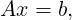(3.1)

here x and b are both column vectors. There are a sequence of already solved linear problems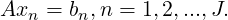(3.2)

Assume xn are all linearly independent. In the successive right-hand method (see ), the best approximation to x is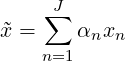(3.3)

which is found by minimizing the object function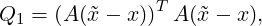(3.4)

or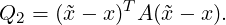(3.5)

If Q1 is used, the projection bases are em = bm,m = 1,2,...,J. Using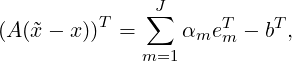(3.6)

there is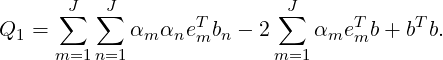(3.7)

To minimize Q1, there should be ∂Q1∕∂αm = 0,m = 1,2,...,J. The corresponding linear problem is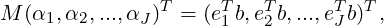(3.8)

with symmetric positive definite coefficient matrix Mmn = emT bn. In this case, both the solutions xm and the right-hand sides bm need to be stored.

If Q2 is used, A should be a symmetric positive definite matrix, as those encountered in the Poisson equation and the Helmholtz equation. Here, the projection bases are êm = xm,m = 1,2,...,J. Using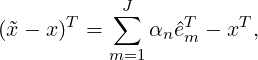(3.9)

there is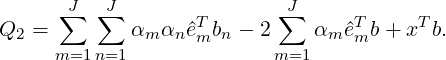(3.10)

To minimize Q2, there should be ∂Q2∕∂αm = 0,m = 1,2,...,J. The corresponding linear problem is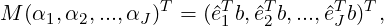(3.11)

with symmetric positive definite coefficient matrix Mmn = êmT bn. In this case, only the solutions xm need to be stored.

The formulations of Q1 version and Q2 version are the same, except the difference of projection bases. By default, Q2 is used as the object function. If you want to use Q1 instead, you can assign a negative value to `SuccessiveRHS`:

1<I PROPERTY="SuccessiveRHS"         VALUE="-8" />

or

1<P> SuccessiveRHS = -8 </P>

In the original paper of Fischer (1998) , the Gram-Schmidt orthogonal process is applied to the projection bases, this method is very stable and avoids the calculation of M-1. However, when the memory space is full, this approach makes it hard to decide which basis should be overwritten. In our implementation, we just store the normalized right-hand sides or old solutions, i.e. emT bm = 1 or êmT bm = 1, and overwrite the oldest ones. The linear problem (3.8) or (3.11) is solved using a direct method.

To make sure M is positive definite, when a new basis eJ+1 arrives, we test the following condition to decide whether or not to accept it,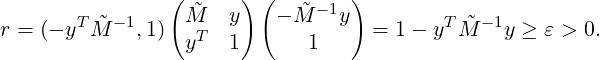(3.12)

Assuming eJ is the oldest basis, there are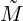mn = Mmn,ym = emT bJ+1,(m,n = 1,2,...,J - 1).

##### 3.3.5.4 PETSc options and configuration

The PETSc solvers, although currently experimental, are operational both in serial and parallel. PETSc gives access to a wide range of alternative solver options such as GMRES, as well as any packages that PETSc can link against, such as the direct multi-frontal solver MUMPS.

Configuration of PETSc options using its command-line interface dictates what matrix storage, solver type and preconditioner should be used. This should be specified in a `.petscrc` file inside your working directory, as command line options are not currently passed through to PETSc to avoid conflict with Nektar++ options. As an example, to select a GMRES solver using an algebraic multigrid preconditioner, and view the residual convergence, one can use the configuration:

-ksp_monitor
-ksp_view
-ksp_type gmres
-pc_type gamg

Or to use MUMPS, one could use the options:

-ksp_type preonly
-pc_type lu
-pc_factor_mat_solver_package mumps
-mat_mumps_icntl_7 2

A final choice that can be specified is whether to use a shell approach. By default, Nektar++ will construct a PETSc sparse matrix (or whatever matrix is specified on the command line). This may, however, prove suboptimal for higher order discretisations. In this case, you may choose to use the Nektar++ matrix-vector operators, which by default use an assembly approach that can prove faster, by setting the `PETScMatMult` `SOLVERINFO` option to `Shell`:

1<I PROPERTY="PETScMatMult" VALUE="Shell" />

The downside to this approach is that you are now constrained to using one of the Nektar++ preconditioners. However, this does give access to a wider range of Krylov methods than are available inside Nektar++ for more advanced users.

#### 3.3.6 Boundary Regions and Conditions

Boundary conditions are defined by two XML elements. The first defines the boundary regions in the domain in terms of composite entities from the `GEOMETRY` section of the file. Each boundary region has a unique ID and are defined as,

1<BOUNDARYREGIONS>
2    <B ID=[id]> [composite-list] </B>
3    ...
4</BOUNDARYREGIONS>

For example,

1<BOUNDARYREGIONS>
2  <B ID="0"> C </B>
3  <B ID="1"> C </B>
4</BOUNDARYREGIONS>

The boundary regions can also optionally contain a name which is then used in the multi-block VTK output to label the block descriptively rather than by ID, for example

1<BOUNDARYREGIONS>
2  <B ID="0" NAME="Wall"> C </B>
3  <B ID="1" NAME="Farfield"> C </B>
4</BOUNDARYREGIONS>

The second XML element defines, for each variable, the condition to impose on each boundary region, and has the form,

1<BOUNDARYCONDITIONS>
2    <REGION REF="[regionID]">
3      <[type1] VAR="[variable1]" VALUE="[expression1]" />
4      ...
5      <[typeN] VAR="[variableN]" VALUE="[expressionN]" />
6    </REGION>
7    ...
8</BOUNDARYCONDITIONS>

There should be precisely one `REGION` entry for each `B` entry defined in the `BOUNDARYREGION` section above. For example, to impose a Dirichlet condition on both variables for a domain with a single region,

1<BOUNDARYCONDITIONS>
2  <REGION REF="0">
3    <D VAR="u" VALUE="sin(PI*x)*cos(PI*y)" />
4    <D VAR="v" VALUE="sin(PI*x)*cos(PI*y)" />
5  </REGION>
6</BOUNDARYCONDITIONS>

Boundary condition specifications may refer to any parameters defined in the session file. The `REF` attribute corresponds to a defined boundary region. The tag used for each variable specifies the type of boundary condition to enforce.

##### 3.3.6.1 Dirichlet (essential) condition

Dirichlet conditions are specified with the `D` tag.

 Projection Homogeneous support Time-dependent support Dimensions CG Yes Yes 1D, 2D and 3D DG Yes Yes 1D, 2D and 3D HDG Yes Yes 1D, 2D and 3D

Example:

1<!-- homogeneous condition -->
2<D VAR="u" VALUE="0" />
3<!-- inhomogeneous condition -->
4<D VAR="u" VALUE="x^2+y^2+z^2" />
5<!-- time-dependent condition -->
6<D VAR="u" USERDEFINEDTYPE="TimeDependent" VALUE="x+t" />

##### 3.3.6.2 Neumann (natural) condition

Neumann conditions are specified with the `N` tag.

 Projection Homogeneous support Time-dependent support Dimensions CG Yes Yes 1D, 2D and 3D DG No No 1D, 2D and 3D HDG ? ? ?

Example:

1<!-- homogeneous condition -->
2<N VAR="u" VALUE="0" />
3<!-- inhomogeneous condition -->
4<N VAR="u" VALUE="x^2+y^2+z^2" />
5<!-- time-dependent condition -->
6<N VAR="u" USERDEFINEDTYPE="TimeDependent" VALUE="x+t" />
7<!-- high-order pressure boundary condition (for IncNavierStokesSolver) -->
8<N VAR="u" USERDEFINEDTYPE="H" VALUE="0" />

##### 3.3.6.3 Periodic condition

Periodic conditions are specified with the `P` tag.

 Projection Homogeneous support Dimensions CG Yes 1D, 2D and 3D DG No 2D and 3D

Example:

1<BOUNDARYREGIONS>
2  <B ID="0"> C </B>
3  <B ID="1"> C </B>
4</BOUNDARYREGIONS>
5
6<BOUNDARYCONDITIONS>
7  <REGION REF="0">
8    <P VAR="u" VALUE="" />
9  </REGION>
10  <REGION REF="1">
11    <P VAR="u" VALUE="" />
12  </REGION>
13</BOUNDARYCONDITIONS>

Periodic boundary conditions are specified in a significantly different form to other conditions. The `VALUE` property is used to specify which `BOUNDARYREGION` is periodic with the current region in square brackets.

Caveats:

• A periodic condition must be set for ”’both”’ boundary regions; simply specifying a condition for region 0 or 1 in the above example is not enough.
• The order of the elements inside the composites defining periodic boundaries is important. For example, if ‘C‘ above is defined as edge IDs ‘0,5,4,3‘ and ‘C‘ as ‘7,12,2,1‘ then edge 0 is periodic with edge 7, 5 with 12, and so on.
• For the above reason, the composites must also therefore be of the same size.
• In three dimensions, care must be taken to correctly align triangular faces which are intended to be periodic. The top (degenerate) vertex should be aligned so that, if the faces were connected, it would lie at the same point on both triangles.
• It is possible specify periodic boundaries that are related by a rotation about a cartesian axis. In three-dimensions it is necessary to specify the rotational arguments to allow the orientation of each periodic face to be determined. This is not required in two-dimensions. An example of how two periodic boundaries are related by a rotation about the x-axis of PI∕6 is shown below. The last number specifies an optional tolerance to which the rotation is considered as equivalent (default value is 1e - 8).
1<BOUNDARYREGIONS>
2  <B ID="0"> C </B>
3  <B ID="1"> C </B>
4</BOUNDARYREGIONS>
5
6<BOUNDARYCONDITIONS>
7  <REGION REF="0">
8    <P VAR="u" USERDEFINEDTYPE="Rotated:x:PI/6:1e-6"  VALUE="" />
9  </REGION>
10  <REGION REF="1">
11    <P VAR="u" USERDEFINEDTYPE="Rotated:x:-PI/6:1e-6"  VALUE="" />
12  </REGION>
13</BOUNDARYCONDITIONS>

##### 3.3.6.4 Time-dependent boundary conditions

Time-dependent boundary conditions may be specified through setting the `USERDEFINEDTYPE` attribute and using the parameter `t` where the current time is required. For example,

1<D VAR="u" USERDEFINEDTYPE="TimeDependent" VALUE="sin(PI*(x-t))" />

##### 3.3.6.5 Boundary conditions from file

Boundary conditions can also be loaded from file. The following example is from the Incompressible Navier-Stokes solver,

1<REGION REF="1">
2  <D VAR="u" FILE="Test_ChanFlow2D_bcsfromfiles_u_1.bc" />
3  <D VAR="v" VALUE="0" />
4  <N VAR="p" USERDEFINEDTYPE="H" VALUE="0" />
5</REGION>

Boundary conditions can also be loaded simultaneously from a file and from an expression (currently only implemented in 3D). For example, in the scenario where a spatial boundary condition is read from a file, but needs to be modulated by a time-dependent expression:

1<REGION REF="1">
2  <D VAR="u" USERDEFINEDTYPE="TimeDependent" VALUE="sin(PI*(x-t))"
3             FILE="bcsfromfiles_u_1.bc" />
4</REGION>

In the case where both `VALUE` and `FILE` are specified, the values are multiplied together to give the final value for the boundary condition.

#### 3.3.7 Functions

Finally, multi-variable functions such as initial conditions and analytic solutions may be specified for use in, or comparison with, simulations. These may be specified using expressions (`<E>`) or imported from a file (`<F>`) using the Nektar++ FLD file format

1<FUNCTION NAME="ExactSolution">
3</FUNCTION>
4<FUNCTION NAME="InitialConditions">
5  <F VAR="u" FILE="session.rst" />
6</FUNCTION>

A restart file is a solution file (in other words an .fld renamed as .rst) where the field data is specified. The expansion order used to generate the .rst file must be the same as that for the simulation. .pts files contain scattered point data which needs to be interpolated to the field. For further information on the file format and the different interpolation schemes, see section 5.6.19. All filenames must be specified relative to the location of the .xml file.

With the additional argument `TIMEDEPENDENT="1"`, different files can be loaded for each timestep. The filenames are defined using boost::format syntax where the step time is used as variable. For example, the function `Baseflow` would load the files `U0V0_1.00000000E-05.fld`, `U0V0_2.00000000E-05.fld` and so on.

1<FUNCTION NAME="Baseflow">
2       <F VAR="U0,V0" TIMEDEPENDENT="1" FILE="U0V0_%14.8E.fld"/>
3</FUNCTION>

For .pts files, the time consuming computation of interpolation weights is only performed for the first timestep. The weights are stored and reused in all subsequent steps, which is why all consecutive .pts files must use the same ordering, number and location of data points.

Other examples of this input feature can be the insertion of a forcing term,

1<FUNCTION NAME="BodyForce">
2  <E VAR="u" VALUE="0" />
3  <E VAR="v" VALUE="0" />
4</FUNCTION>
5<FUNCTION NAME="Forcing">
6  <E VAR="u" VALUE="-(Lambda + 2*PI*PI)*sin(PI*x)*sin(PI*y)" />
7</FUNCTION>

or of a linear advection term

2  <E VAR="Vx" VALUE="1.0" />
3  <E VAR="Vy" VALUE="1.0" />
4  <E VAR="Vz" VALUE="1.0" />
5</FUNCTION>

##### 3.3.7.1 Remapping variable names

Note that it is sometimes the case that the variables being used in the solver do not match those saved in the FLD file. For example, if one runs a three-dimensional incompressible Navier-Stokes simulation, this produces an FLD file with the variables `u`, `v`, `w` and `p`. If we wanted to use this velocity field as input for an advection velocity, the advection-diffusion-reaction solver expects the variables `Vx`, `Vy` and `Vz`. We can manually specify this mapping by adding a colon to the filename, indicating the variable names in the target file that align with the desired function variable names. This gives a definition such as:

2  <F VAR="Vx,Vy,Vz" FILE="file.fld:u,v,w" />
3</FUNCTION>

There are some caveats with this syntax:

• The same number of fields must be defined for both the `VAR` attribute and in the comma-separated list after the colon. For example, the following is not valid:
2  <F VAR="Vx,Vy,Vz" FILE="file.fld:u" />
3</FUNCTION>
• This syntax is not valid with the wildcard operator `*`, so one cannot write for example:
2  <F VAR="*" FILE="file.fld:u,v,w" />
3</FUNCTION>

##### 3.3.7.2 Time-dependent file-based functions

With the additional argument `TIMEDEPENDENT="1"`, different files can be loaded for each timestep. The filenames are defined using boost::format syntax where the step time is used as variable. For example, the function `Baseflow` would load the files `U0V0_1.00000000E-05.fld`, `U0V0_2.00000000E-05.fld` and so on.

1<FUNCTION NAME="Baseflow">
2  <F VAR="U0,V0" TIMEDEPENDENT="1" FILE="U0V0_%14.8R.fld" />
3</FUNCTION>

Section 3.7 provides the list of acceptable mathematical functions and other related technical details.

#### 3.3.8 Quasi-3D approach

To generate a Quasi-3D appraoch with Nektar++ we only need to create a 2D or a 1D mesh, as reported above, and then specify the parameters to extend the problem to a 3D case. For a 2D spectral/hp element problem, we have a 2D mesh and along with the parameters we need to define the problem (i.e. equation type, boundary conditions, etc.). The only thing we need to do, to extend it to a Quasi-3D approach, is to specify some additional parameters which characterise the harmonic expansion in the third direction. First we need to specify in the solver information section that that the problem will be extended to have one homogeneouns dimension; here an example

1<SOLVERINFO>
2  ...
3  <I PROPERTY="HOMOGENEOUS"           VALUE="1D"                       />
4</SOLVERINFO>

then we need to specify the parameters which define the 1D harmonic expanson along the z-axis, namely the homogeneous length (LZ) and the number of modes in the homogeneous direction (HomModesZ). `HomModesZ` corresponds also to the number of quadrature points in the homogenous direction, hence on the number of 2D planes discretized with a spectral/hp element method.

1<PARAMETERS>
2  ...
3  <P> HomModesZ     = 4       </P>
4  <P> LZ            = 1.0     </P>
5</PARAMETERS>

In case we want to create a Quasi-3D approach starting from a 1D spectral/hp element mesh, the procedure is the same, but we need to specify the parameters for two harmonic directions (in Y and Z direction). For Example,

1<SOLVERINFO>
2  ...
3  <I PROPERTY="HOMOGENEOUS"           VALUE="2D"                         />
4</SOLVERINFO>
5<PARAMETERS>
6  ...
7  <P> HomModesY     = 10    </P>
8  <P> LY            = 6.5   </P>
9  <P> HomModesZ     = 6     </P>
10  <P> LZ            = 2.0   </P>
11</PARAMETERS>

By default the operations associated with the harmonic expansions are performed with the Matrix-Vector-Multiplication (MVM) defined inside the code. The Fast Fourier Transform (FFT) can be used to speed up the operations (if the FFTW library has been compiled in ThirdParty, see the compilation instructions). To use the FFT routines we need just to insert a flag in the solver information as below:

1<SOLVERINFO>
2  ...
3  <I PROPERTY="HOMOGENEOUS"           VALUE="2D"                         />
4  <I PROPERTY="USEFFT"                VALUE="FFTW"                       />
5</SOLVERINFO>

The number of homogeneous modes has to be even. The Quasi-3D approach can be created starting from a 2D mesh and adding one homogenous expansion or starting form a 1D mesh and adding two homogeneous expansions. Not other options available. In case of a 1D homogeneous extension, the homogeneous direction will be the z-axis. In case of a 2D homogeneous extension, the homogeneous directions will be the y-axis and the z-axis.